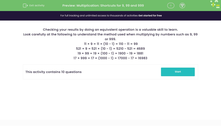# Multiplication: Shortcuts for 9, 99 and 999

In this worksheet, students develop their mental arithmetic skills by using shortcuts to help them multiply quickly and accurately.Key stage:  KS 3

Curriculum topic:   Number

Curriculum subtopic:   Use Four Operations for All Numbers

Difficulty level:#### Worksheet Overview

Checking your results by doing an equivalent operation is a valuable skill to learn.

Look carefully at the following to understand the method used when multiplying by numbers such as 9, 99 or 999.

11 × 9 = 11 × (10 - 1) = 110 - 11 = 99

521 × 9 = 521 × (10 - 1) = 5210 - 521 = 4689

19 × 99 = 19 × (100 - 1) = 1900 - 19 = 1881

17 × 999 = 17 × (1000 - 1) = 17000 - 17 = 16983

### What is EdPlace?

We're your National Curriculum aligned online education content provider helping each child succeed in English, maths and science from year 1 to GCSE. With an EdPlace account you’ll be able to track and measure progress, helping each child achieve their best. We build confidence and attainment by personalising each child’s learning at a level that suits them.

Get started